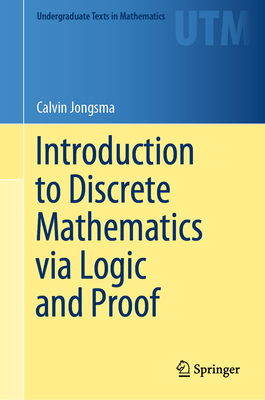# Introduction to Discrete Mathematics Via Logic and Proof (Undergraduate Texts in Mathematics)

Current price: \$59.99
Publication Date: December 12th, 2019
Publisher:
Springer
ISBN:
9783030253578
Pages:
482
The MIT Press Bookstore
1 on hand, as of Dec 8 10:45am
(MATH)
On Our Shelves Now

## Description

This textbook introduces discrete mathematics by emphasizing the importance of reading and writing proofs. Because it begins by carefully establishing a familiarity with mathematical logic and proof, this approach suits not only a discrete mathematics course, but can also function as a transition to proof. Its unique, deductive perspective on mathematical logic provides students with the tools to more deeply understand mathematical methodology--an approach that the author has successfully classroom tested for decades.

Chapters are helpfully organized so that, as they escalate in complexity, their underlying connections are easily identifiable. Mathematical logic and proofs are first introduced before moving onto more complex topics in discrete mathematics. Some of these topics include:

• Mathematical and structural induction
• Set theory
• Combinatorics
• Functions, relations, and ordered sets
• Boolean algebra and Boolean functions
• Graph theory

Introduction to Discrete Mathematics via Logic and Proof will suit intermediate undergraduates majoring in mathematics, computer science, engineering, and related subjects with no formal prerequisites beyond a background in secondary mathematics.